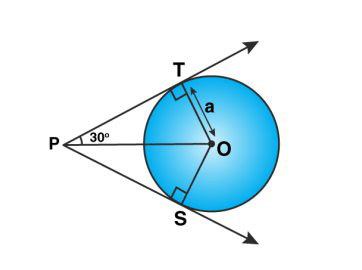# RS Aggarwal Solutions Class 10 Mathematics Solutions for Circles Exercise 8B in Chapter 8 - Circles

Question 6 Circles Exercise 8B

If the angle between two tangents drawn from an external point P to a circle of radius and center O is 60° then find the length of OP.

Since tangents are drawn from an external point are equally inclined to the line joining center to that point. Draw an image based on given instructions:Here, PT is the tangent of the circle OT ⊥ PT And, ∠TPS = 60° (given) ∠TPO = 60°/2 = 30° Now, from right ∆TPO: sin 30° = OT/OP 1/2 = a/OP or OP = 2a

Related Questions

Lido

Courses

Teachers

Book a Demo with us

Syllabus

Maths
CBSE
Maths
ICSE
Science
CBSE

Science
ICSE
English
CBSE
English
ICSE
Coding

Terms & Policies

Selina Question Bank

Maths
Physics
Biology

Allied Question Bank

Chemistry
Connect with us on social media!